# Down in the depths ‘on’ Carathéodory’s theorem

Research in general, and mathematical one in particular, sometimes reminds to the lyrics that Cole Porter wrote for the Down in the depths song:

And here am I

Facing tomorrow

Alone with my sorrow

Down in the depths on the ninetieth floor.

Nevertheless, sometimes you manage to get down in the depths and achieve a nice and/or remarkable result. The paper consider here achieves both, by extending a classical theorem in a clever and interesting way.

First things first, convex geometry is a branch of mathematics which, despite dating back from ancient times, has boosted particularly in the last century. Its main object of study are those sets being closed under convex combinations, called convex sets. In the more familiar setting of a d-dimensional Euclidean space (like the 2D-plane or the 3D-space), convex sets are then those for which the straight segment joining any two points is entirely contained in the set. The following figure shows examples of convex (left) and non-convex (right) sets.

Focusing on discrete convex geometry, an outstanding notion is that of the convex hull, or convex envelope, of a given set of points in the d-dimensional space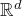. This is the smallest convex set containing the points, and its computation is, for example, a central problem in computational geometry. Informally, for the 2D case we can see the convex hull as the result obtained if we surround the point set with an expanded rubber band, and then release it allowing its contraction.

This simple notion of convex hull is the main ingredient for one of the outstanding classical theorems in convex geometry, proved by Carathéodory 1 in 1907, which states that

For any finite set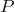of points in, given any point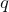contained in the convex hull of, there exist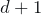points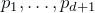insuch thatis contained in their convex hull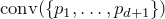.

For the two-dimensional case, with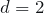, this means that given any point“inside” an arbitrary set of points, there are 3 points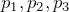that containinside the triangle they define. (Note that general position, i.e., no three points aligned, should be assumed for the triangle to be proper.) The result might seem trivial for this 2D case but be aware that geometry in higher dimensions is not so apparent and many counterintuitive situations arise.

Back to intuitions, have a look at the left and right configurations in the following figure: Which of the two possibilities for(red point) do you think is contained in the larger number of triangles spanned by points in(black)?

You probably have guessed that the configuration on the left should have more triangles containingthan the configuration on the right: In the one on the left, choosing one point in each of the three blades of the windmill gives rise to a triangle containing, that is, there are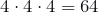such triangles. However, in the one on the right, since the pointis just an epsilon over the lower edge of the triangular envelope, that edge is forced to be part of any triangle containing, and actually the only possibilities are the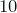triangles using that edge plus an additional point.

In view of the previous example, it seems that the deeper isinside, the larger number of triangles can be chosen containing. Formalizing this intuition is the main result in a recent paper by Fabila-Monroy and Huemer 2 who, 110 years later than the original paper by Carathéodory, have succeeded to prove the following result:

For any finite setof points in, given any pointof positive depth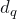with respect to, there is a constant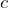depending onand there existpairwise disjoint subsets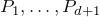of, each of them with cardinality at least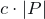, such that, for every choice of a point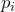in each of the subsets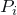, the pointis contained in the convex hullof the chosen points.

Informally, this statement says that the deeper isinside, the larger subsetsexist such that choosing one point in each of them gives an envelope containing. In this sense, the original and classical Carathéodory’s theorem states the existence of setswith cardinality one, while this recent result goes further by ensuring certain cardinality which depends on the depth. In the left configuration of the example above, we have already seen three subsets of cardinality 4 as in the statement, namely the three blades of the windmill.

There are actually many possibilities to define how deep is a pointinside the envelope of a point set. The notion of depth considered by Fabila-Monroy and Huemer is the so-called Tukey depth, defined as the minimum number of points fromin a halfplane containing. In the example above, the depth ofis 4 for the left configuration and 2 for the right configuration.

As their key ingredient, Ruy-Fabila and Huemer introduce the notion of projection Tukey depth of a pointwith respect to a setand a hyperplane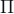. Informally, a large projection Tukey depth means that there exists a direction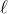in whichcan be projected onto hyperplanes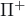and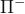, parallel toon opposite sides, such that in the resulting configurations the pointhas large Tukey depth with respect to the projections of.

Defining the projection Tukey depth of a pointwith respect to a setas the minimum over all the possible hyperplanes, the authors prove a projection-depth version of the well-known centerpoint theorem (related to the geometric analogous of the median):

Given any finite setof points in, there exists a point, not necessarily in, whose projection Tukey depth with respect tois at least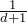.

Last, but not least, the authors also prove depth versions of two other classical theorems, namely Helly’s 3 and Kirchberger’s 4. As a summary, this work allows to revisit several classical and important theorems, providing at the same time a new and enriched perspective which enhances their deepness.

Note: This text is part of the dissemination activities of the Marie Skłodowska-Curie grant No 734922 in the European Union’s Horizon 2020 research and innovation programme.

## References

1. Carathéodory, C. (1907). Über den Variabilitätsbereich der Koeffizienten von Potenzreihen, die gegebene Werte nicht annehmen. Mathematische Annalen, 64(1), 95-115. doi:10.1007/BF01449883
2. Fabila-Monroy, R. & Huemer, C. (2017). Discrete and Computational Geometry, 58(1): 51-66. doi: 10.1007/s00454-017-9893-8
3. Danzer, L., Grünbaum, B., and Klee, V. (1963). Helly’s theorem and its relatives. AMS
4. Kirchberger, P. (1903). Über Tchebychefsche Annäherungsmethoden. Mathematische Annalen, 57(4), 509-540. doi: 10.1007/BF01445182